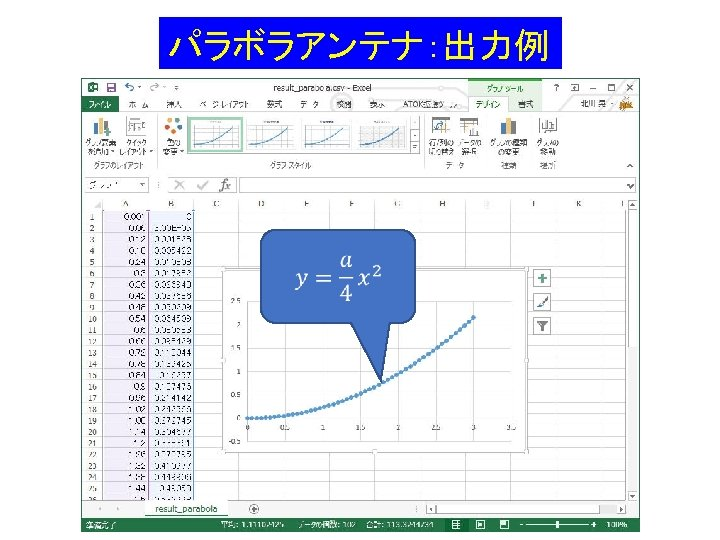# Dim Writer As New IO Stream Writer C

• Slides: 13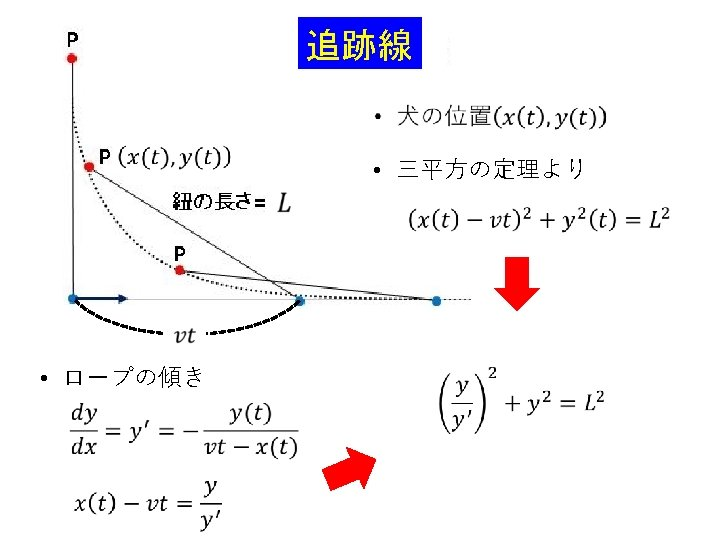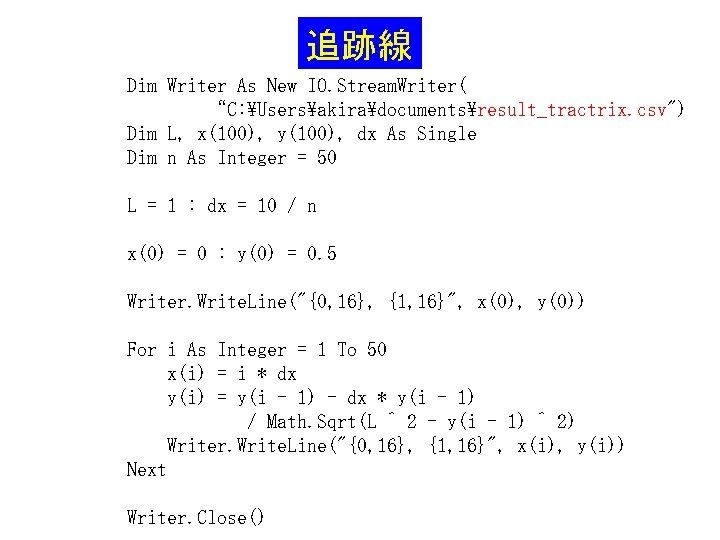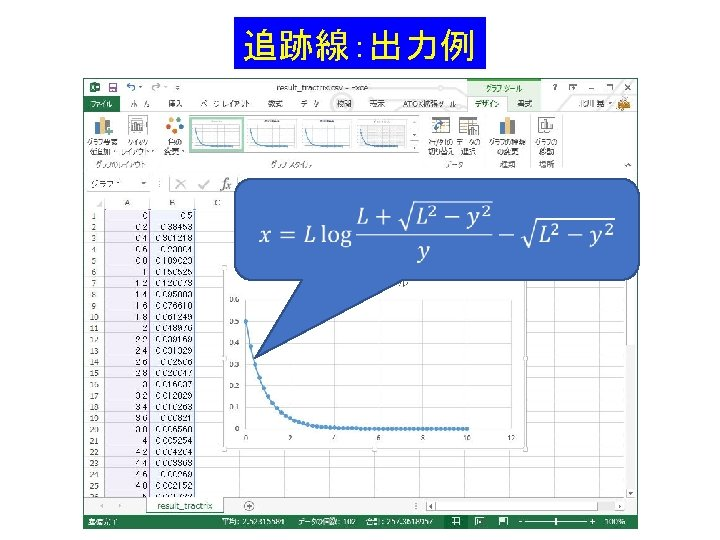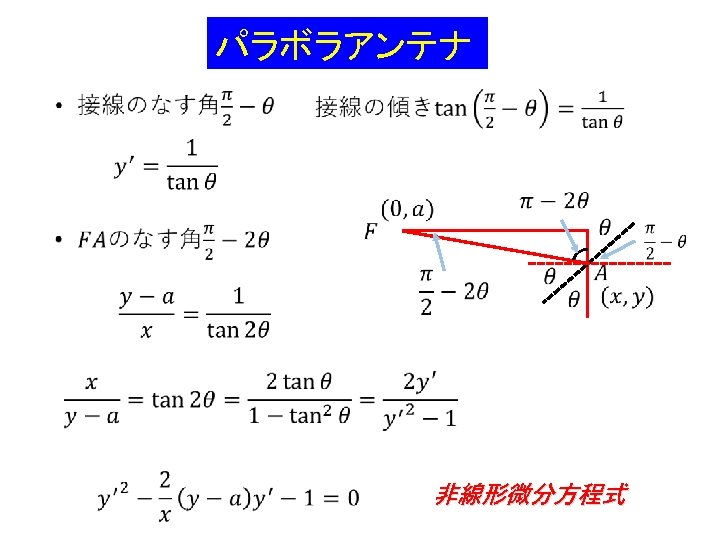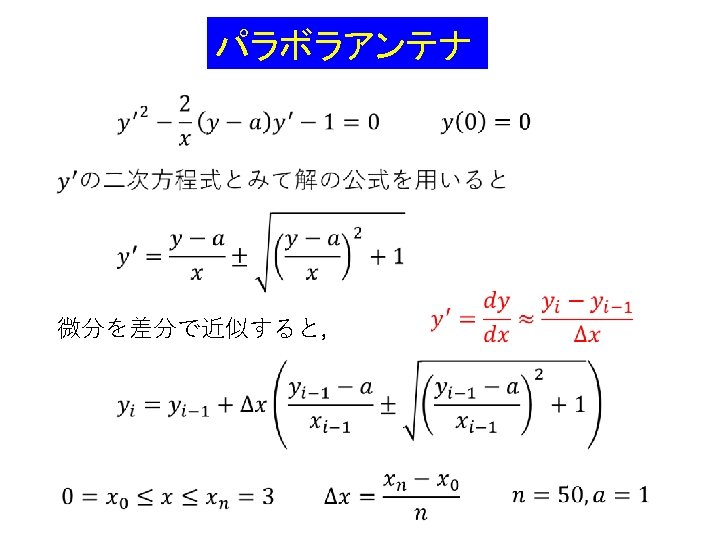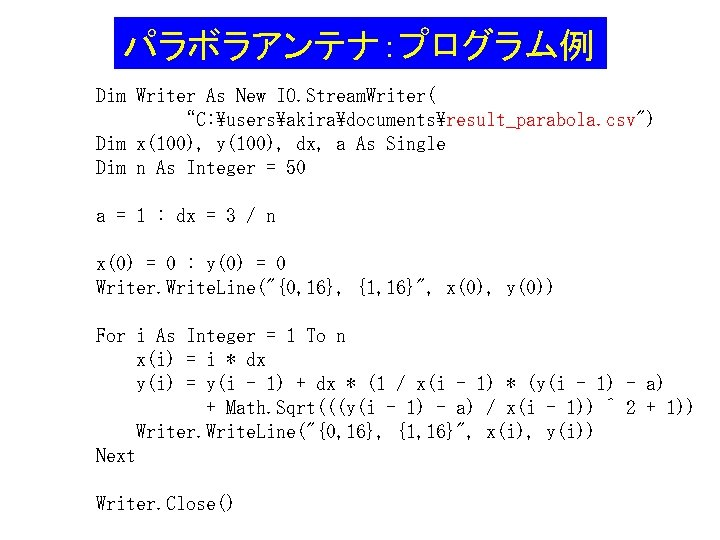パラボラアンテナ：プログラム例 Dim Writer As New IO. Stream. Writer( “C: usersakiradocumentsresult_parabola. csv") Dim x(100), y(100), dx, a As Single Dim n As Integer = 50 a = 1 : dx = 3 / n x(0) = 0 : y(0) = 0 Writer. Write. Line("{0, 16}, {1, 16}", x(0), y(0)) For i As Integer = 1 To n x(i) = i * dx y(i) = y(i - 1) + dx * (1 / x(i - 1) * (y(i - 1) - a) + Math. Sqrt(((y(i - 1) - a) / x(i - 1)) ^ 2 + 1)) Writer. Write. Line("{0, 16}, {1, 16}", x(i), y(i)) Next Writer. Close()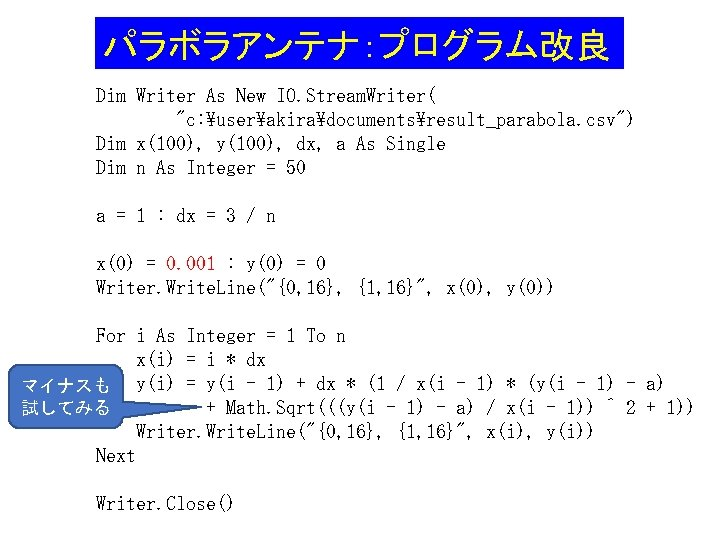パラボラアンテナ：プログラム改良 Dim Writer As New IO. Stream. Writer( "c: userakiradocumentsresult_parabola. csv") Dim x(100), y(100), dx, a As Single Dim n As Integer = 50 a = 1 : dx = 3 / n x(0) = 0. 001 : y(0) = 0 Writer. Write. Line("{0, 16}, {1, 16}", x(0), y(0)) For i As Integer = 1 To n x(i) = i * dx マイナスも y(i) = y(i - 1) + dx * (1 / x(i - 1) * (y(i - 1) - a) + Math. Sqrt(((y(i - 1) - a) / x(i - 1)) ^ 2 + 1)) 試してみる Writer. Write. Line("{0, 16}, {1, 16}", x(i), y(i)) Next Writer. Close()